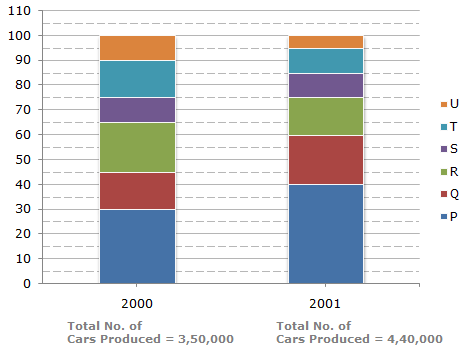# Data Interpretation - Bar Charts - Discussion

Discussion Forum : Bar Charts - Bar Chart 9 (Q.No. 3)
Directions to Solve

The bar graph given below shows the percentage distribution of the total production of a car manufacturing company into various models over two years.

Percentage of Six different types of Cars manufactured by a Company over Two Years3.
If the percentage production of P type cars in 2001 was the same as that in 2000, then the number of P type cars produced in 2001 would have been?
1,40,000
1,32,000
1,17,000
1,05,000
Explanation:

If the percentage production of P type cars in 2001

= Percentage production of P type cars in 2000

= 30%.

then, number of P type cars produced in 2001

= 30% of 4,40,000

= 1,32,000.

Discussion:
7 comments Page 1 of 1.

Manpreet Kaur 7 A said:   2 years ago
Because P type car manufactured in 2000 was 30%.

Sravani said:   4 years ago
Please anyone can explain to me how come 30%?

Andhi said:   6 years ago
According to me, this problem have no answer.

When we choose 132000 as the answer the total of cars produced will less than 440000 or the exact number = (100%-40%)x440000 + 132000 = 396000.

And 30% of 396000 = 118800. so 132000 is not 30% of total cars produced in 2001 (it is 33,3%).

To find the right number so P type cars produced in 2001 have exact 30% of all company in 2001 below are the equation.
(100%-40%)x440000 + 30%xA = A.

We must find the total cars produced (A). The answer A = 377142,8571. 30% of A = 113142,8571. So the answer should 113142,8571.

Sultan said:   7 years ago
@Yamini : 30% of 4,40,000 = (440000/100) * 30 = 4400 * 30 = 132000.

Sultan said:   7 years ago
@Abhinav, because we need to calculate the cars produced in 2001.

Abhinav shrivastava said:   8 years ago
Why we multiplied by 4, 40000 if we have to calculate the car for year 2000 which is same as for the year 2001?

Yamini.pinnapothu said:   9 years ago
Please any one can explain me how the 30% get?